# incremental 3D convex hull

Tags: , , , ,for those who don’t know, a convex hull is the tightest volume enclosing a given set of vertices so that none of them lies outside the volume. it can be useful in some cases, like when you want to perform coarse collision detection on a complex volume.

very soon I’ll attend the Flash On The Beach for the second time, last year I was lucky enough to show something during the elevator pitch and now I’m going as an entusiastic attendee.
time to meet up with some good friends, hang around with talented bastards and get shit faced for 4 days :)

I’d like to stress the fact that this year’s elevator pitch is going to break some jaws ; I’ve heard that simo would be there along with devboy and the psykopaint dude.

last year, my pitch was about 3D and stuff. I wanted to break apart 3D objects on the fly (a dynamic fracture algorithm ), so I had to do a 3D convex hull. it was fun, it gave me a chance to study the Half edge data structure, a nifty little tool used for computational geometry.
as I was not doing much yesterday I thought I’d rewrite the hulling algo from scratch, 3D-engine free (for the pitch I used minko, so my implementation was strongly coupled to it ), only with the native API .

here’s a demo: click to respawn, when the hulling time is 0, you can click again to respawn the thing.

it’s very heavy to render as the full convex hull is computed onEnterframe, it’s just to give you the idea.

I used the incremental version, the slowest and the easiest to implement.
the reason why I’m sharing this is that I was pretty pleased with the processing speed.
of course there’s plenty of room for optimisation, the first tetrahedra computation is a bit weird for instance and could be done better I guess. also the Face creation could be inlined and stored otherwise, it’s a tradeoff I did for convenience mostly.

on my computer, it takes about 60-70ms ms in the debug player for a thousand points which is way (way way way) faster than my previous attempts. you’ll find the source code and a sample call at the bottom of the page but before that I’d like to show you a couple of outputs ( one of the reasons I did this is also because I find it beautiful :) )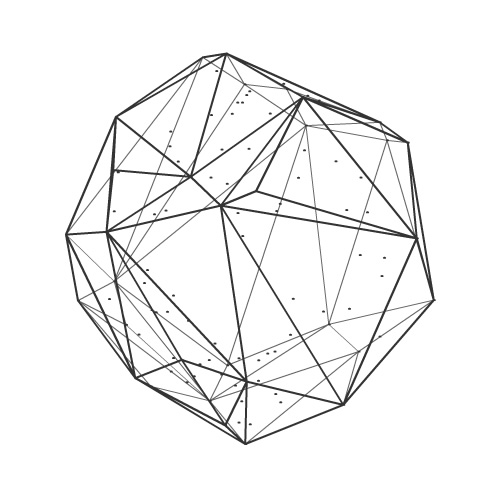for a 100 vector3Dfor a 1000 vector3D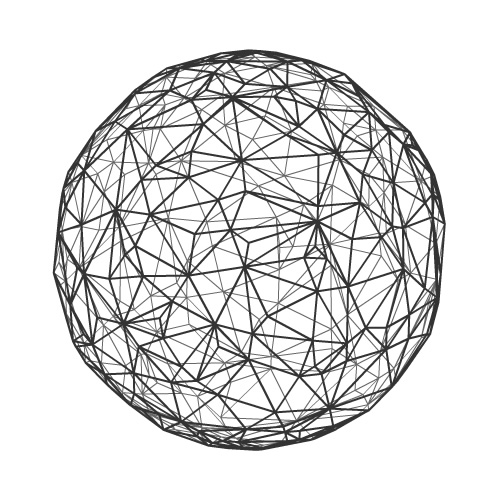for 10.000 vector3D (not rendering the dots anymore, too many of them)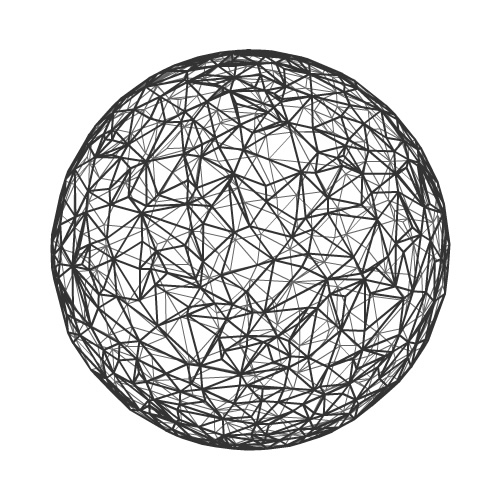for 50.000 vector3D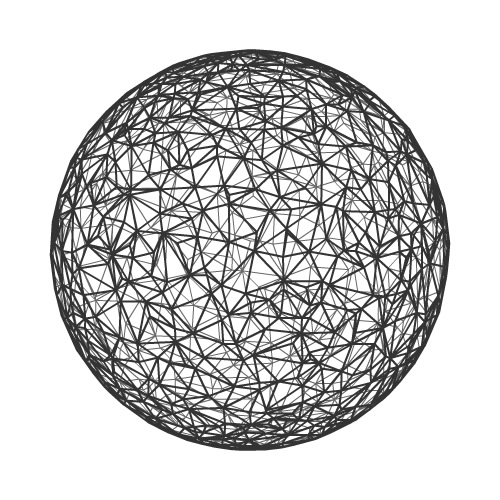and finally 100.000 vector3D at this point it becomes absurd : use a bounding sphere :)

here’s another distribution like a cube or something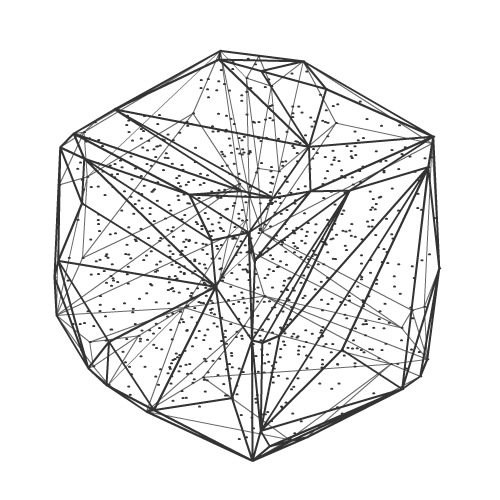with 1000 points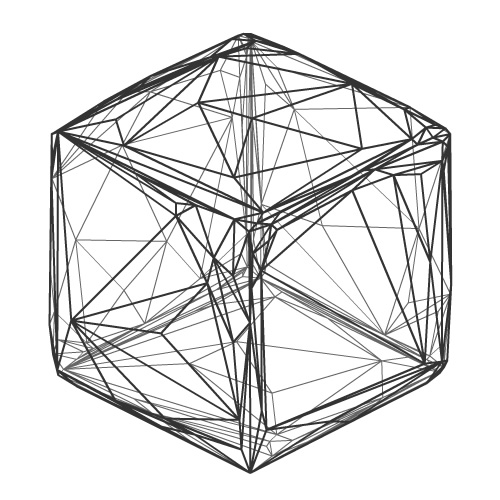with 50000 points
again, if you have this kind of distributions, then use a bounding box.

here’s the source code: 3D convex hull

and here’s a sample code:

```private function reset():void
{

var unprojected:Vector.<Number> = new Vector.<Number>();
var vertices:Vector.<Number> = new Vector.<Number>();
var uvs:Vector.<Number> = new Vector.<Number>();

//create some points
var points:Vector.<Vector3D> = new Vector.<Vector3D>();
var size:Number = 200;
var i:int = 1000;
while ( i-- )
{
points.push( randomVector( size ) );
}

//convex hulling
var indices:Vector.<int> = ConvexHull.process( points );

//rendering
for each ( var v:Vector3D in points )
{
unprojected.push( v.x, v.y, v.z );
}
Utils3D.projectVectors( new Matrix3D(), unprojected, vertices, uvs );

graphics.lineStyle( 1 );
graphics.drawTriangles( vertices, indices, uvs );

}
//randomly creates a vertice on a sphere
private function randomVector( offset:Number ):Vector3D
{

var radius:Number =  ( .5 + Math.random() * .5 ) * offset;

var theta:Number = Math.random() * 2 * Math.PI;
var phi:Number = Math.random() * Math.PI;

return new Vector3D( 	Math.cos( theta ) * Math.sin( phi ) * radius,
Math.sin( theta ) * Math.sin( phi ) * radius );
}```

this should give you something like below: I’ve added a click to respawn the Vertices and the rotation.

when the mouse is in the center, the rendering stops, it spares lots of resources.

that was it,
enjoy :)

Tags: , , , ,